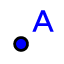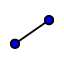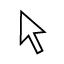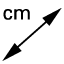# Exploring the relationships between different lengths in a circle.

Topic:
Circle

## Instructions

Part 1: Exploring the properties of the radii of a circle.
1. Mark three points, A, B and C on the circle using.
2. Using, draw a line from the centre O to points, A, B and C respectively.
3. Measure the radius OA, OB and OC using.
4. What do you notice about the length between each of these three points and the centre O?
5. Dragthe red dot to change the size of the circle.
6. What do you notice about the radii as the size of the circle changes?
Part 2: Exploring the properties of the Diameter of a circle.
1. Using, draw a line from the red dot to green dot.
2. Measure the diameter using.
3. Compare it with the radius in part 1.
4. What do you notice about the diameter of the circle and its radius?
5. Dragthe red dot to change the size of the circle.
6. What do you notice about the diameter of the circle as the size of the circle increases?